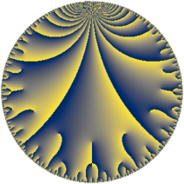Properties

 Label 91.10.cLevel $91$ Weight $10$ Character orbit 91.c Rep. character $\chi_{91}(64,\cdot)$ Character field $\Q$ Dimension $62$ Newform subspaces $1$ Sturm bound $93$ Trace bound $0$

Related objects

Defining parameters

 Level: $$N$$ $$=$$ $$91 = 7 \cdot 13$$ Weight: $$k$$ $$=$$ $$10$$ Character orbit: $$[\chi]$$ $$=$$ 91.c (of order $$2$$ and degree $$1$$) Character conductor: $$\operatorname{cond}(\chi)$$ $$=$$ $$13$$ Character field: $$\Q$$ Newform subspaces: $$1$$ Sturm bound: $$93$$ Trace bound: $$0$$

Dimensions

The following table gives the dimensions of various subspaces of $$M_{10}(91, [\chi])$$.

Total New Old
Modular forms 86 62 24
Cusp forms 82 62 20
Eisenstein series 4 0 4

Trace form

 $$62 q - 15360 q^{4} + 378842 q^{9} + O(q^{10})$$ $$62 q - 15360 q^{4} + 378842 q^{9} - 129080 q^{10} - 86340 q^{12} + 212162 q^{13} - 153664 q^{14} + 4327304 q^{16} + 1652452 q^{17} - 5012396 q^{22} - 1278096 q^{23} - 9442150 q^{25} - 10907340 q^{26} + 16794384 q^{27} - 10363760 q^{29} + 8275336 q^{30} - 8902908 q^{35} - 157163812 q^{36} + 7473860 q^{38} - 45113592 q^{39} + 6264340 q^{40} - 12446784 q^{42} + 112595748 q^{43} - 235575712 q^{48} - 357417662 q^{49} + 76280072 q^{51} + 184926780 q^{52} + 163068704 q^{53} - 171698952 q^{55} + 118013952 q^{56} + 528120292 q^{61} + 327058744 q^{62} - 1108215168 q^{64} + 430577400 q^{65} + 1069507908 q^{66} - 760577284 q^{68} - 154846856 q^{69} - 2010119892 q^{74} + 881911824 q^{75} + 19918696 q^{77} + 251736268 q^{78} + 114047552 q^{79} + 50540630 q^{81} - 3357122608 q^{82} - 7796519536 q^{87} + 6249329764 q^{88} + 2721868364 q^{90} + 601248816 q^{91} + 6449180076 q^{92} - 5238038284 q^{94} + 504345292 q^{95} + O(q^{100})$$

Decomposition of $$S_{10}^{\mathrm{new}}(91, [\chi])$$ into newform subspaces

Label Dim. $$A$$ Field CM Traces $q$-expansion
$a_{2}$ $a_{3}$ $a_{5}$ $a_{7}$
91.10.c.a $62$ $46.868$ None $$0$$ $$0$$ $$0$$ $$0$$

Decomposition of $$S_{10}^{\mathrm{old}}(91, [\chi])$$ into lower level spaces

$$S_{10}^{\mathrm{old}}(91, [\chi]) \cong$$ $$S_{10}^{\mathrm{new}}(13, [\chi])$$$$^{\oplus 2}$$